2017-10-12 22:47:01 yxp520123 阅读数 147
• ###### 机器学习数学基础之矩阵视频教学

本课程囊括了机器学习理论中所需要的和线性代数相关的所有知识。帮助想入门机器学习的朋友们快速掌握数学基础。 主要内容： 1. 矩阵的定义、性质、运算、分解以及应用。 2. 线性空间、范数、生成子空间相关知识。

11637 人正在学习 去看看 AI100讲师

L0范数的定义:

L1范数的定义:

L2范数的定义:

1）特征选择(Feature Selection)：

2）可解释性(Interpretability)：

L2范数是凸函数,可以刻画相似度,预测值和标记值的距离问题,并且L2范数可以作为参数矩阵的正则化项,让参数矩阵尽可能平滑,避免过拟合。

L2范数是指向量各元素的平方和然后求平方根。我们让L2范数的规则项||W||2最小，可以使得W的每个元素都很小，都接近于0，但与L1范数不同，它不会让它等于0，而是接近于0，这里是有很大的区别的哦。而越小的参数说明模型越简单，越简单的模型则越不容易产生过拟合现象。为什么越小的参数说明模型越简单？我也不懂，我的理解是：限制了参数很小，实际上就限制了多项式某些分量的影响很小（看上面线性回归的模型的那个拟合的图），这样就相当于减少参数个数。

L2范数的好处是什么呢？这里也扯上两点：

1）学习理论的角度：

2）优化计算的角度：

2019-11-07 17:15:29 NEW_old_WEI 阅读数 30
• ###### 机器学习数学基础之矩阵视频教学

本课程囊括了机器学习理论中所需要的和线性代数相关的所有知识。帮助想入门机器学习的朋友们快速掌握数学基础。 主要内容： 1. 矩阵的定义、性质、运算、分解以及应用。 2. 线性空间、范数、生成子空间相关知识。

11637 人正在学习 去看看 AI100讲师

## 机器学习NO.3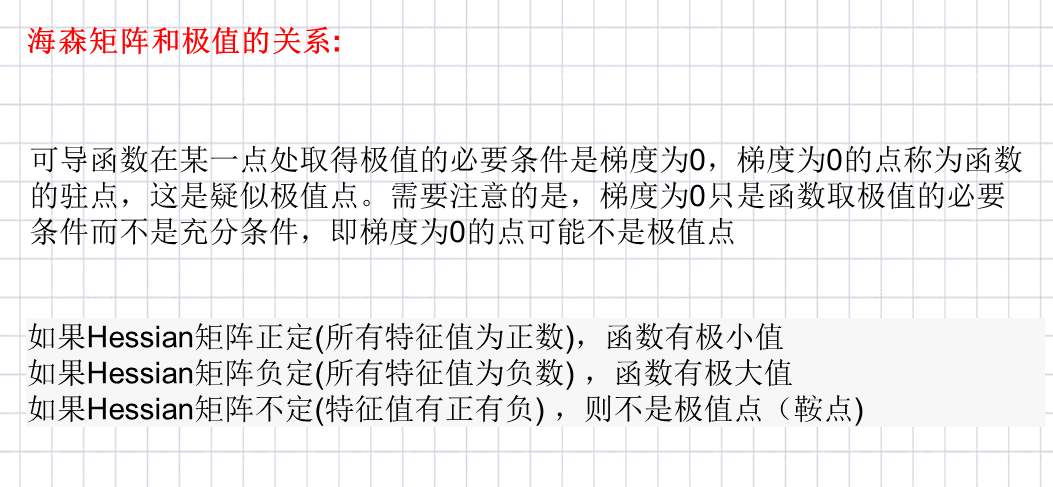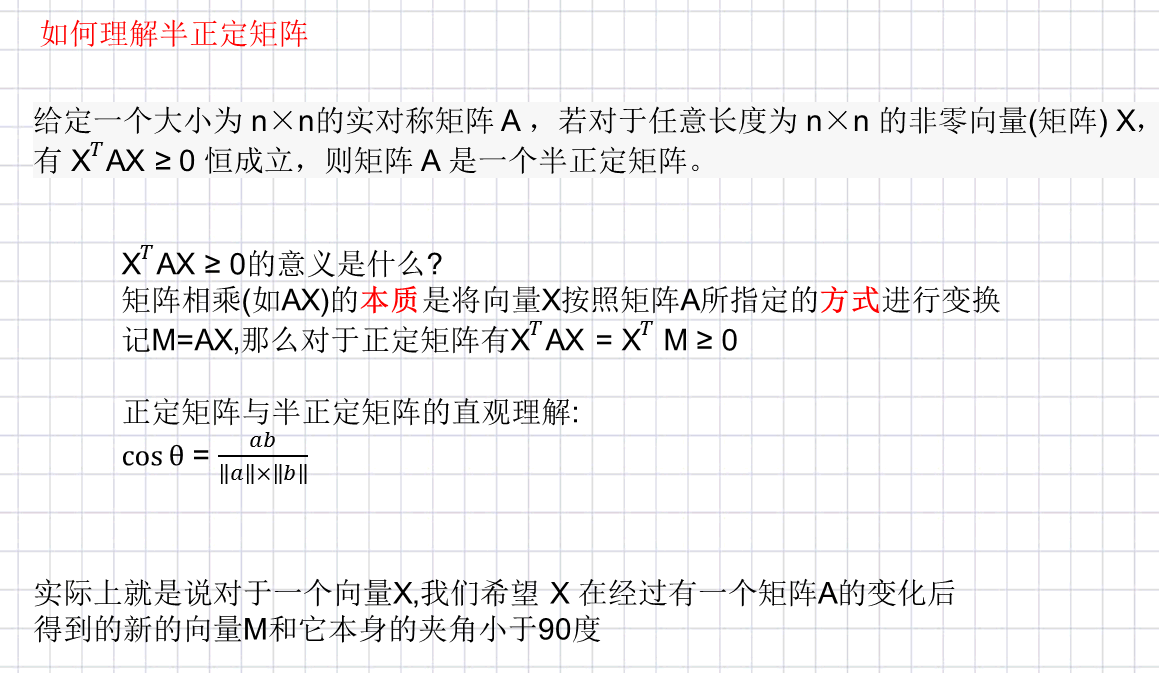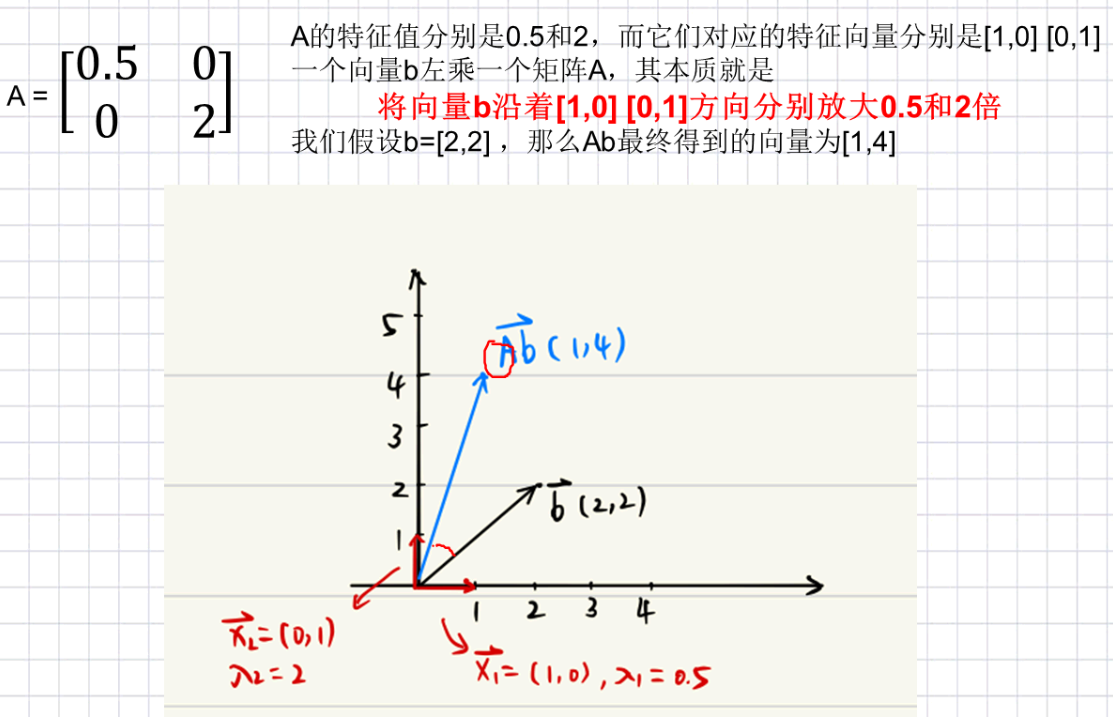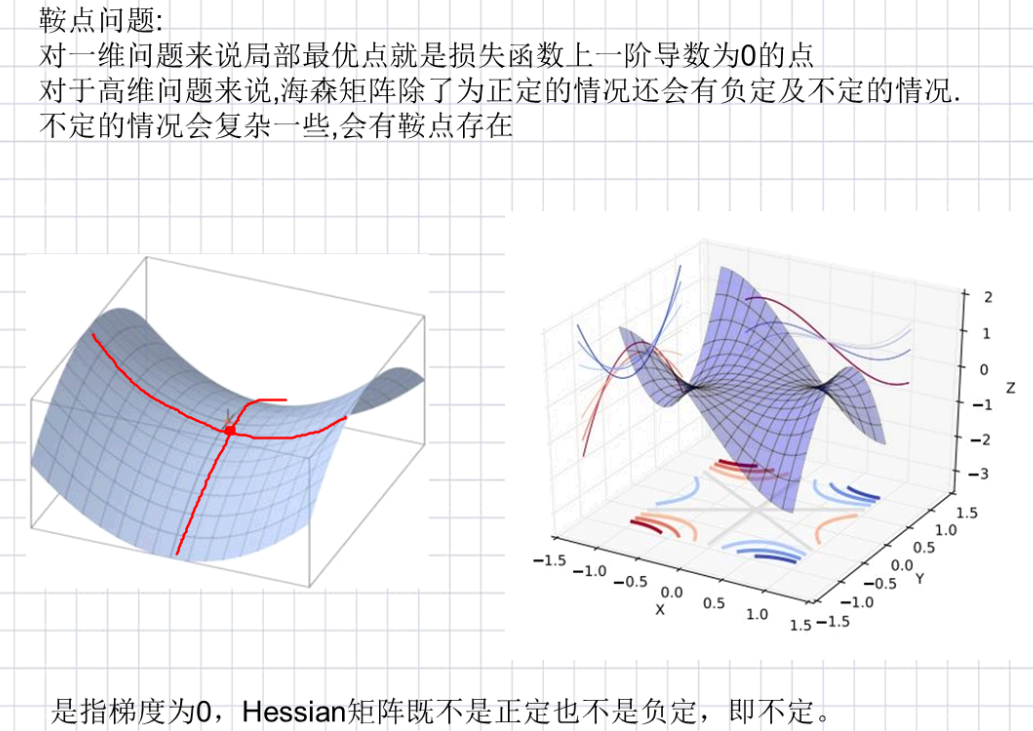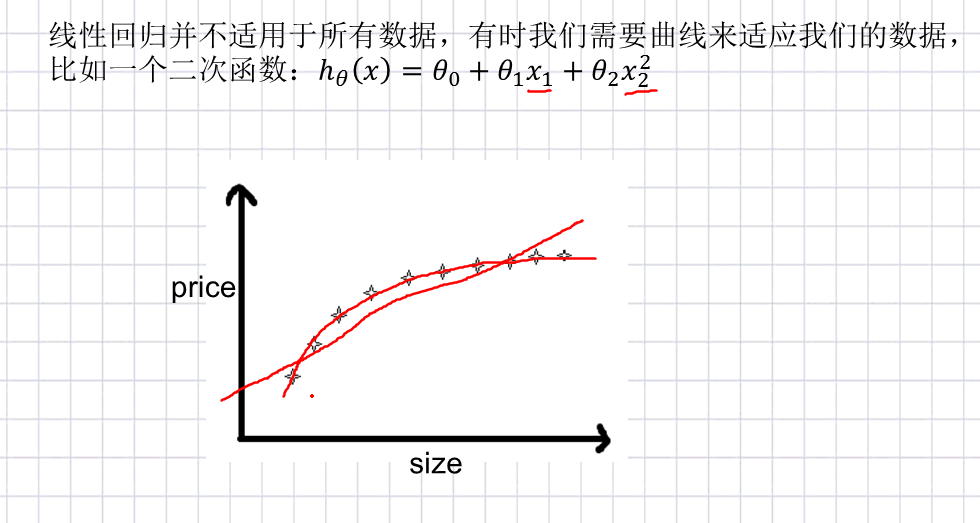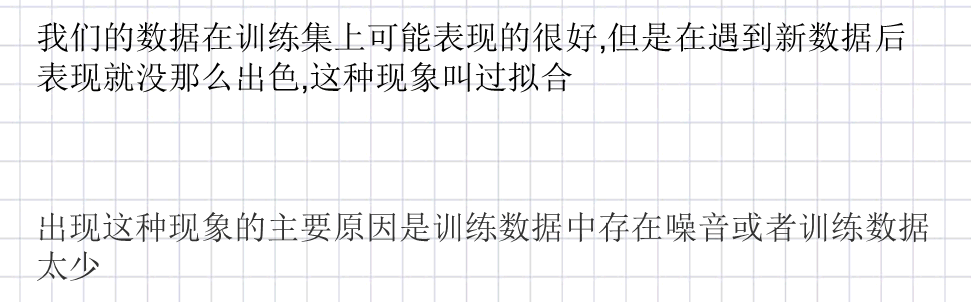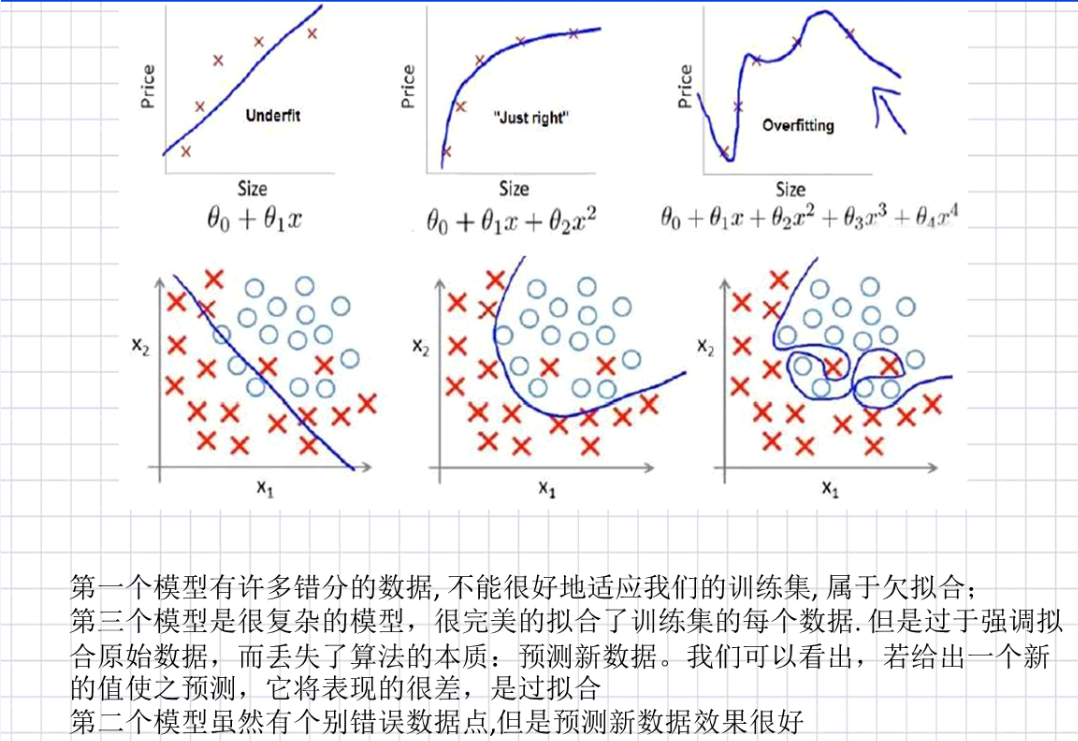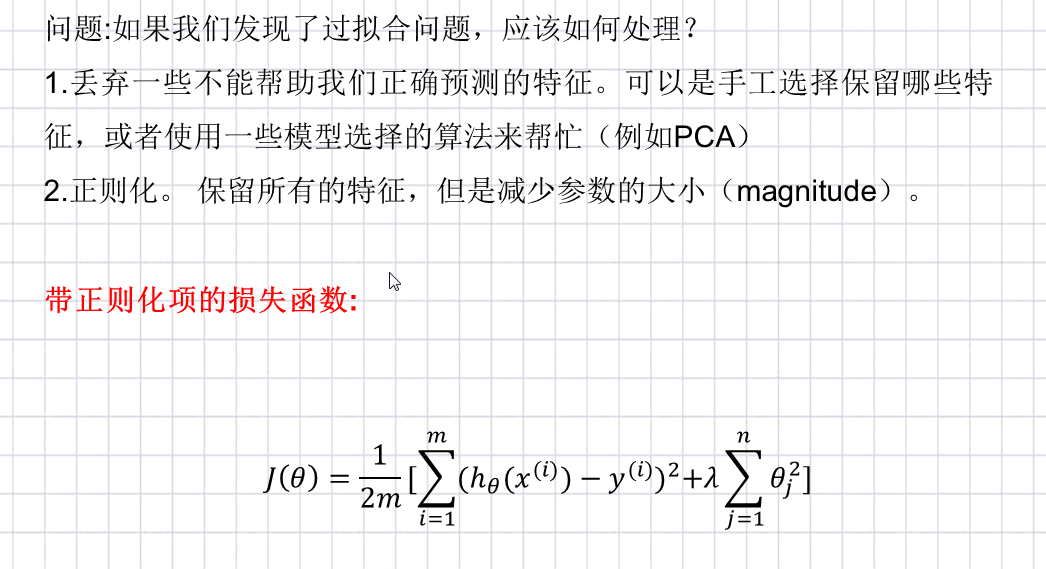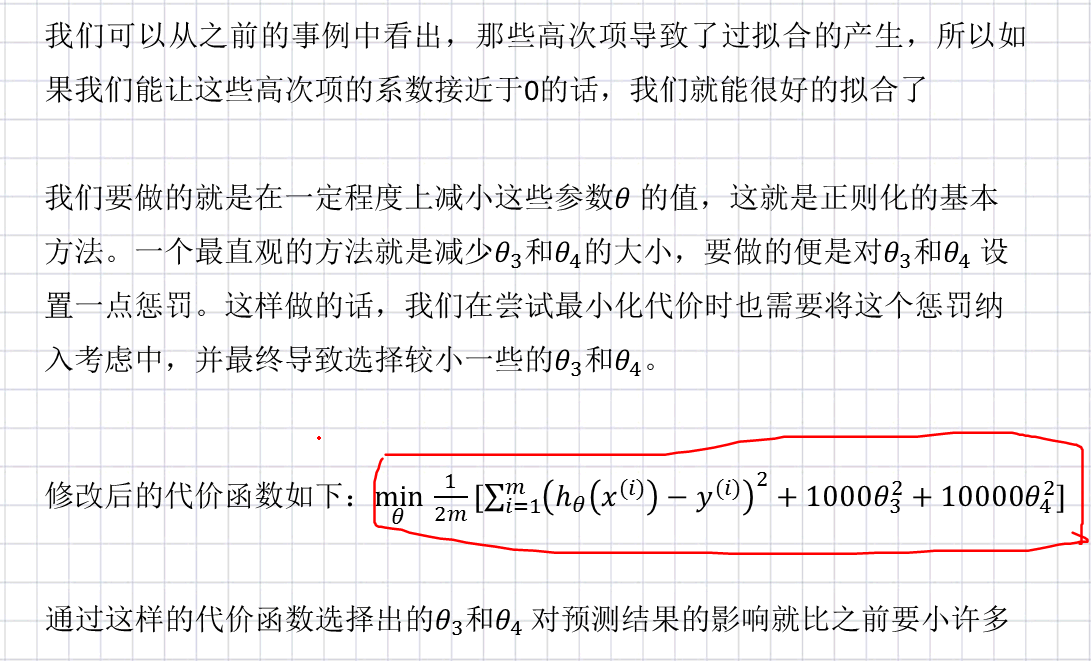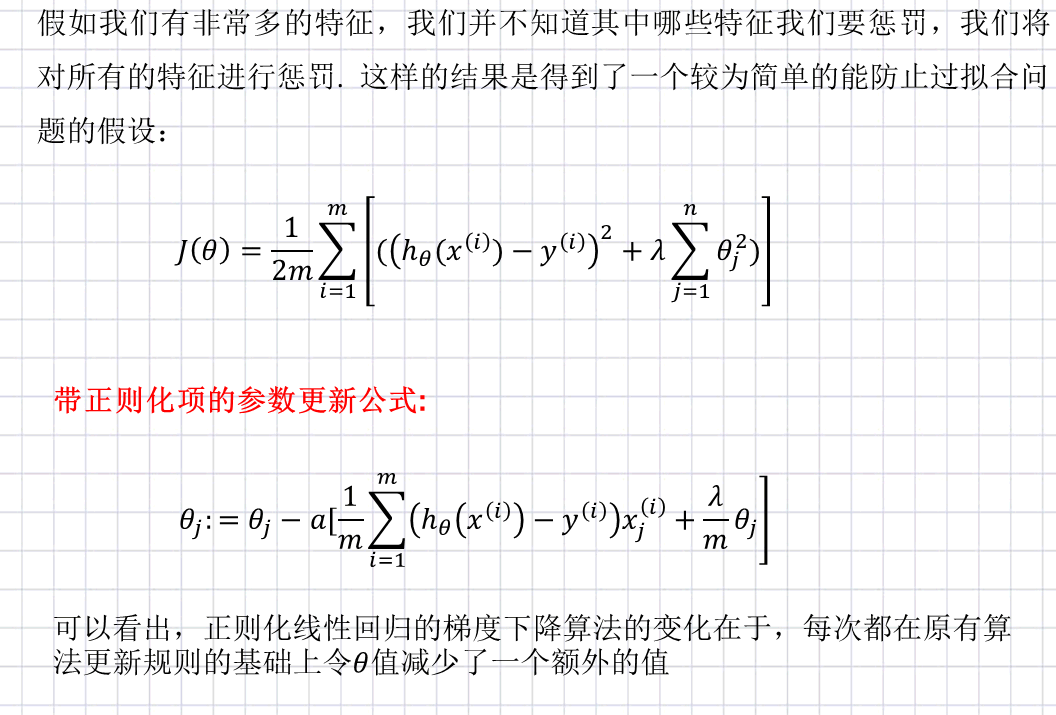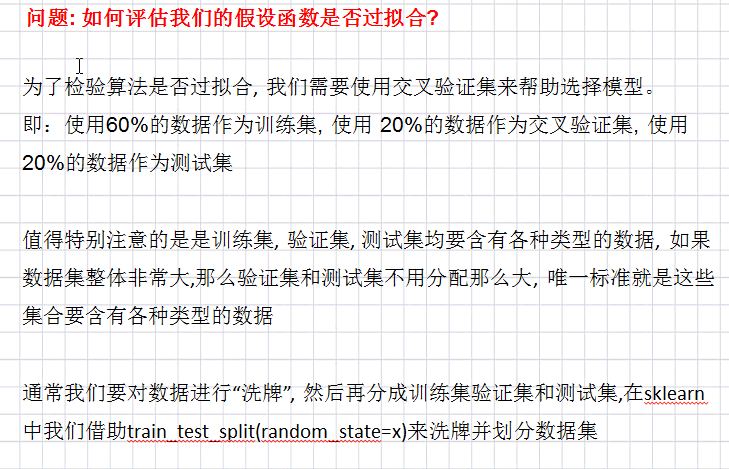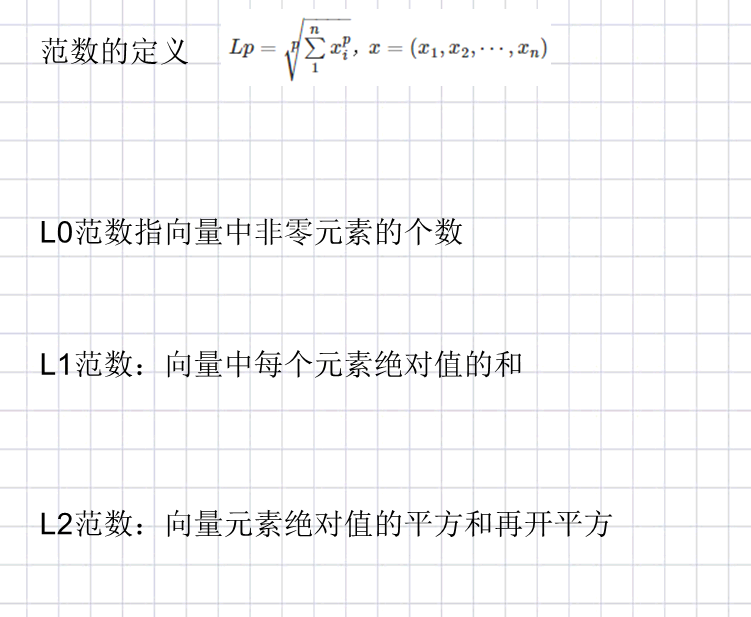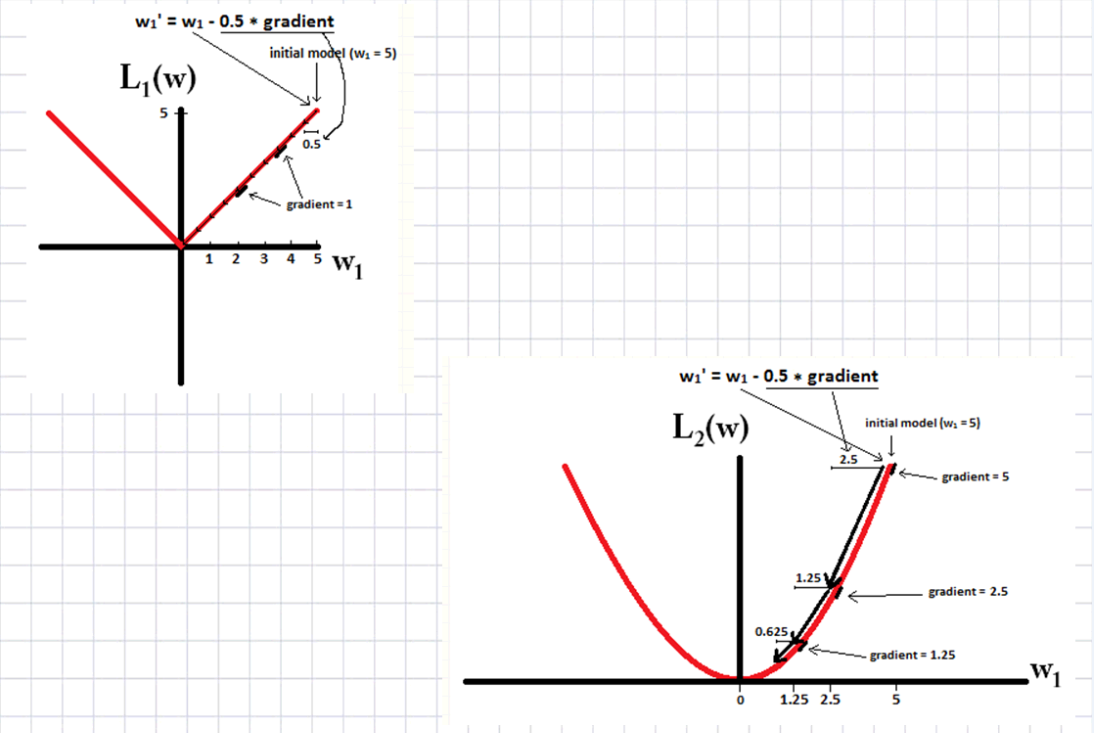## L1正则和L2正则的区别：

1.L1是模型各个参数的绝对值之和。
2.L2是模型各个参数的平方和的开方值。
3.L1会趋向于产生少量特征，而其他的特征都是0，因为最优的参数值很大概率出现在坐标轴上，这样就会导致某一维的权重为0，产生稀疏权重矩阵
4.L2会选择更多的特征，这些特征都会接近于0.最优的参数值很小概率出现在坐标轴上，因此每一维的参数都不会是0.当最小化||w||时，就会是每一项趋近于0

## 机器学习问题解决流程（初学者）

1.用少量数据金额简单模型跑出一个baseline
2.绘制学习曲线，检验是否有高偏差和高方差
3.根据学习曲线来决定下一步行动，更多的数据或者更多的特征
4.人工检查验证集中算法预测错误的实例，尝试找到其中的共性来着受优化
5.尝试其他模型

## 多项式回归Polynomial regressio

``````import numpy as np
import os
import matplotlib.pyplot as plt
#画图
%matplotlib inline

#随机种子
np.random.seed(42)

#保存图像
PROJECT_ROOT_DIR="."
lujing="第三天"

def save_fig(fig_id,tight_layout=True):#定义一个保存图像的函数
path = os.path.join(PROJECT_ROOT_DIR,"images",lujing,fig_id+".png")#指定保存图像路径 当前目录下的images文件夹下的qwertyuio文件夹
print("Saving figure",fig_id)#提示函数，正在保存图片
plt.savefig(path,format='png',dpi=300)#保存图片（需要指定保存路径，保存格式，清晰度）

#把讨厌的警告信息过滤掉
import warnings
warnings.filterwarnings(action="ignore",message="^internal gelsd")

m=100
X=6*np.random.rand(m,1)-3#创建数据集的特征部分
y=0.5*X**2+X+2+np.random.rand(m,1)#创建数据集的标签部分

plt.plot(X,y,"b.")#把数据点画出来
plt.xlabel("x_1",fontsize=18)
plt.ylabel("y",rotation=0,fontsize=18)
plt.axis([-3,3,0,10])
save_fig("一图")#保存图像
plt.show()
``````

Saving figure 一图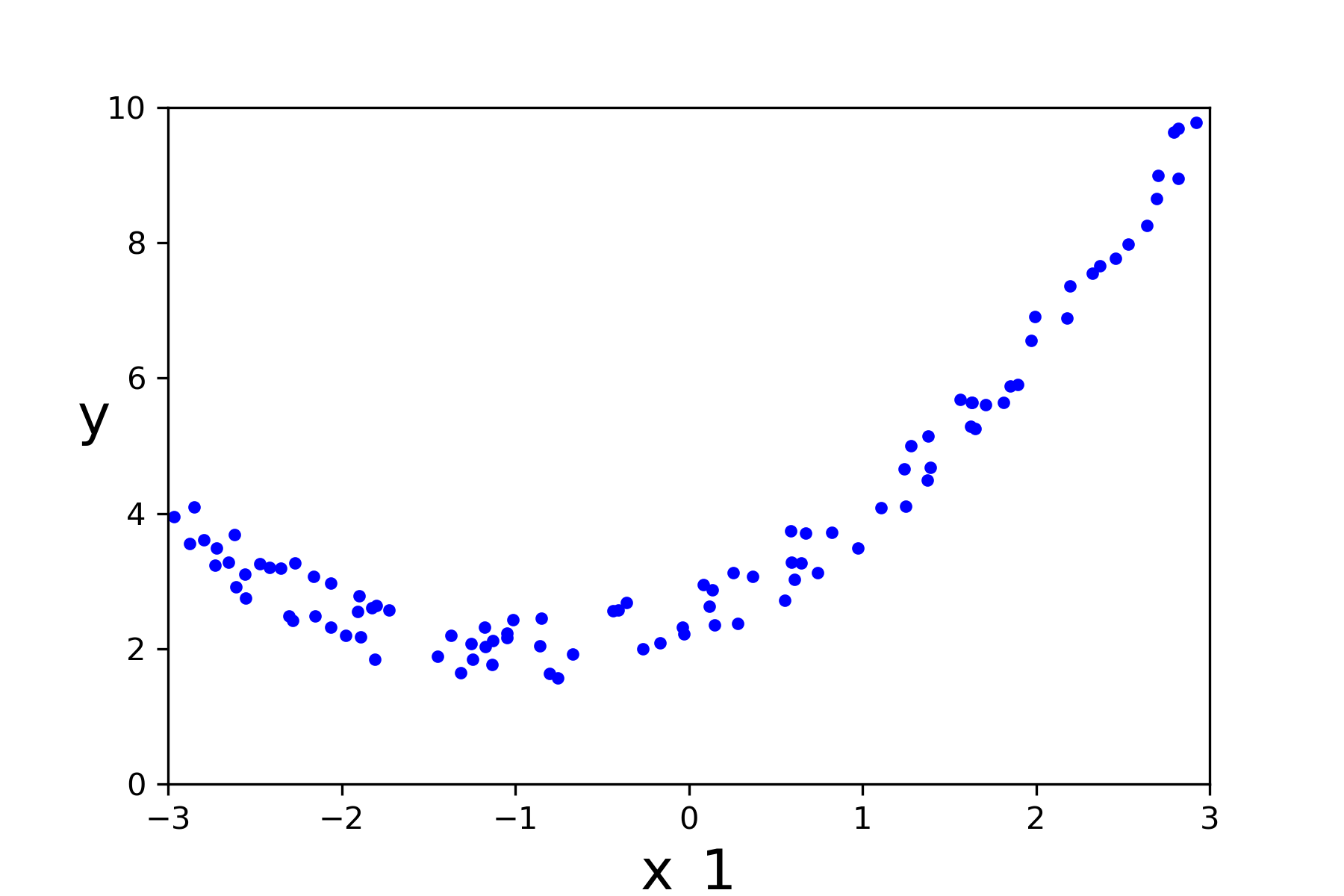``````from sklearn.preprocessing import PolynomialFeatures#从预处理包中导出多项式特征处理模块
from sklearn.linear_model import LinearRegression#从线性模型里导入夏宁分类器

poly_features=PolynomialFeatures(degree=2,include_bias=False)#初始化多项式特征对象
X_poly = poly_features.fit_transform(X)#转化原始特征为多项式特征
poly_features
``````

PolynomialFeatures(degree=2, include_bias=False, interaction_only=False,
order=‘C’)

``````lin_reg = LinearRegression()#初始化一个线性分类器
lin_reg.fit(X_poly,y)#分类器拟合多项特征
lin_reg.intercept_,lin_reg.coef_#获取参数
``````

(array([2.49786712]), array([[0.9943591 , 0.49967213]]))

``````X_new = np.linspace(-3,3,100).reshape(100,1)
X_new_poly = poly_features.transform(X_new)
y_new=lin_reg.predict(X_new_poly)
plt.plot(X,y,"b.")
plt.plot(X_new,y_new,"r-",linewidth=2,label="Predictions")
plt.xlabel("x_1",fontsize=18)
plt.ylabel("y",rotation=0,fontsize=18)
plt.legend(loc="upper left",fontsize=14)
plt.axis([-3,3,0,10])
save_fig("二图")
plt.show()
``````

Saving figure 二图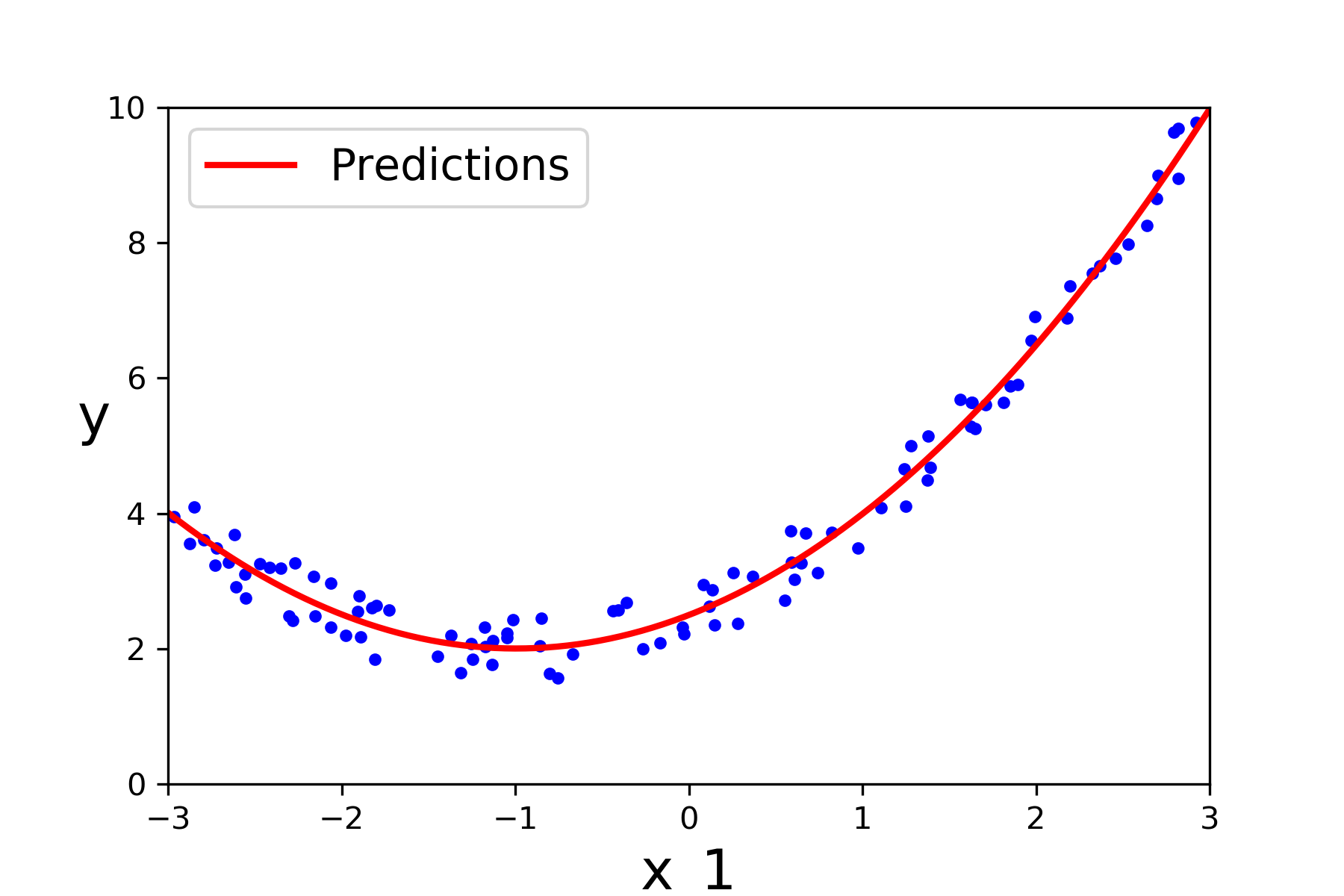``````from sklearn.preprocessing import StandardScaler#从预览处理包里导入标准化处理模块
from sklearn.preprocessing import MinMaxScaler#从预览处理包中导入归一处理模块
from sklearn.pipeline import Pipeline#导入流水先处理模块

for style,width,degree in (("g-",1,300),("b--",2,2),("r-+",2,1)):#遍历三种画图方式
polybig_features = PolynomialFeatures(degree=degree,include_bias=False)#初始化多项式特征对象
std_scaler = StandardScaler()#数据标准化
mm_scaler = MinMaxScaler()
lin_reg = LinearRegression()#初始化线性分类器
polynomial_regression = Pipeline([
("poly_features",polybig_features),#pipeline第一步 处理特征
("std_scaler",std_scaler),#标准化数据
("lin_reg",lin_reg),#初始化线性回归器
])
polynomial_regression.fit(X,y)#训练模式
y_newbig = polynomial_regression.predict(X_new)#预测数据
plt.plot(X_new,y_newbig,style,label=str(degree),linewidth=width)#画拟合好的3个曲线中的一个

plt.plot(X,y,"b.",linewidth=3)#画原始数据点
plt.legend(loc="upper left")
plt.xlabel("x_1",fontsize=18)
plt.ylabel("y",rotation=0,fontsize=18)
plt.axis([-3,3,0,10])
save_fig("三图")
plt.show()

``````

Saving figure 三图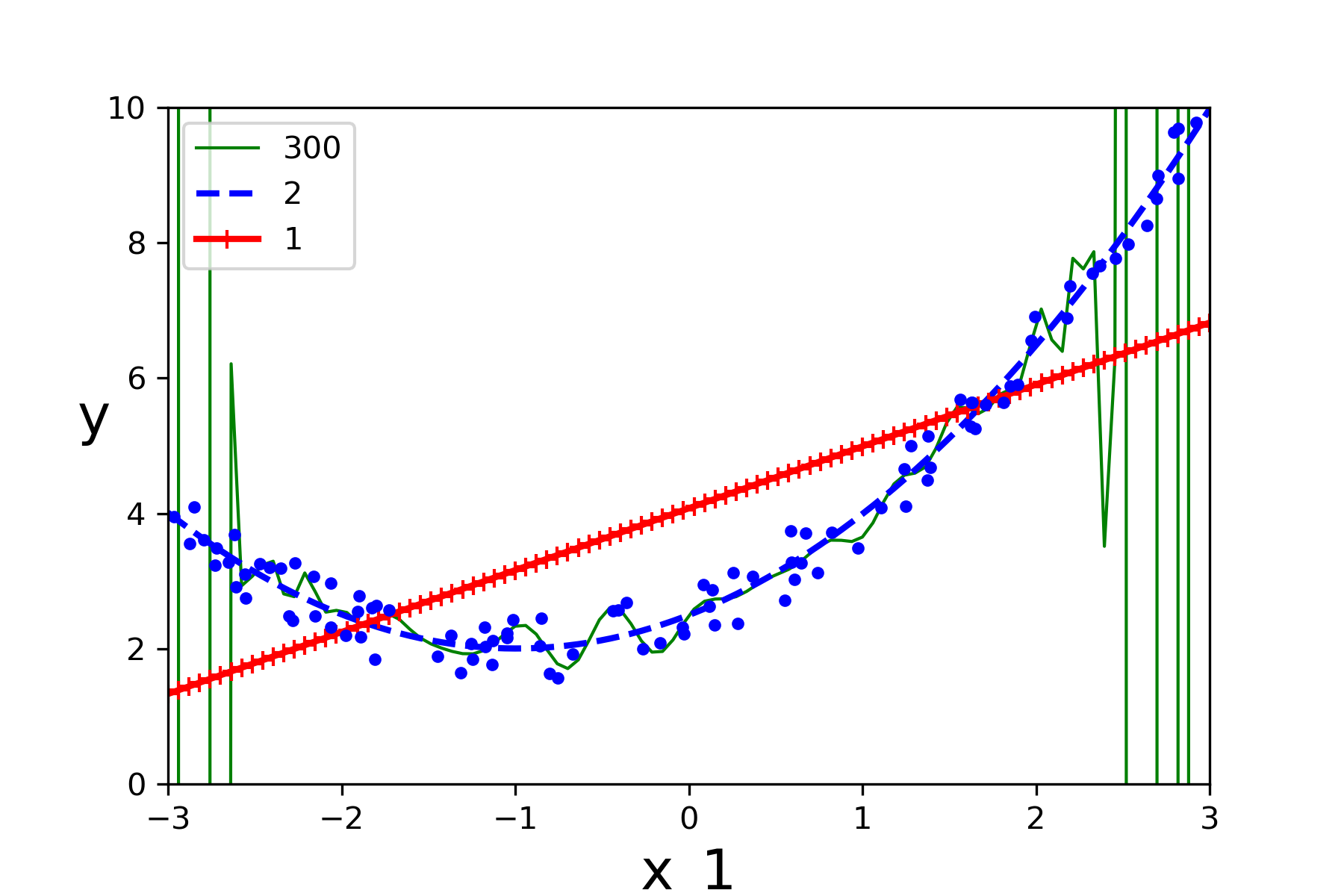## 学习曲线

``````from sklearn.metrics import mean_squared_error#从度量包里导入均方误差的衡量标准
from sklearn.model_selection import train_test_split#从模型选择包里导入数据集切分模块

def plot_learning_curves(model,X,y):
X_train,X_val,y_train,y_val = train_test_split(X,y,test_size=0.2,random_state=6)#切分数据集，训练集80%。验证集20%，指定随即种子
train_errors=[]#收集训练误差
val_errors=[]#收集验证误差
for m in range(1,len(X_train)):#遍历训练集数据
model.fit(X_train[:m],y_train[:m])#拟合数据
y_train_predict = model.predict(X_train[:m])#预测训练集的值
y_val_predict=model.predict(X_val)#预测验证集的值
train_errors.append(mean_squared_error(y_train[:m],y_train_predict))#计算真实值和训练集预测值之间的均方误差并收集
val_errors.append(mean_squared_error(y_val,y_val_predict))#计算真实值和验证集预测值之间的均方误差并收集

plt.plot(np.sqrt(train_errors),"r-+",linewidth=2,label="train")
plt.plot(np.sqrt(val_errors),"b-",linewidth=3,label="val")
plt.legend(loc="upper right",fontsize=14)   #not shown i the book
plt.xlabel("Training set size",fontsize=14) #not shown
plt.ylabel("RMSE",fontsize=14)              #not shown

lin_reg = LinearRegression()#初始化线性回归器
plot_learning_curves(lin_reg,X,y)#调用函数
plt.axis([0,80,0,3])                           #not shown i the book
save_fig("四图")                               #not shown
plt.show()                                     #not shown
``````

Saving figure 四图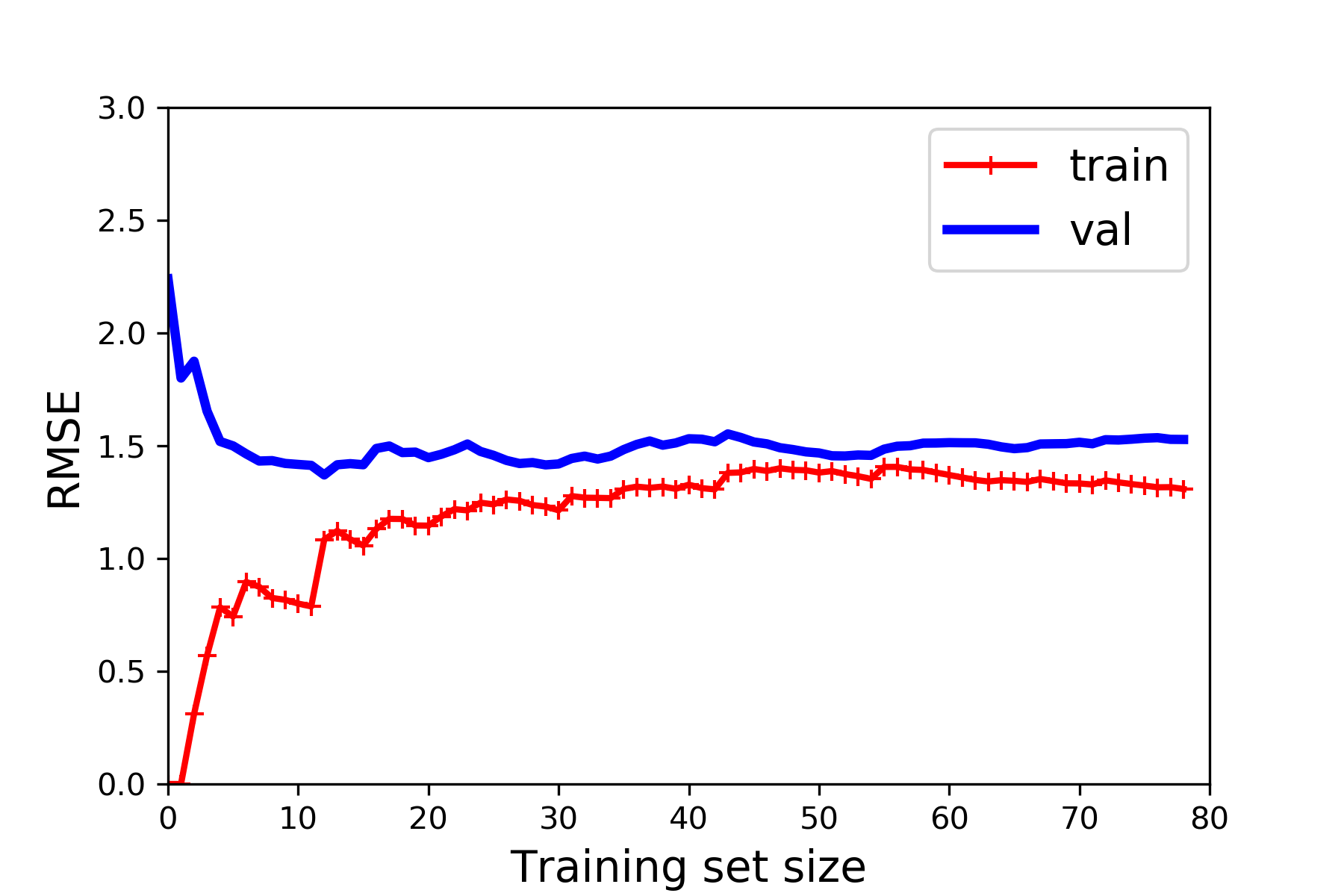2019-05-02 16:25:10 qq_36536126 阅读数 82
• ###### 机器学习数学基础之矩阵视频教学

本课程囊括了机器学习理论中所需要的和线性代数相关的所有知识。帮助想入门机器学习的朋友们快速掌握数学基础。 主要内容： 1. 矩阵的定义、性质、运算、分解以及应用。 2. 线性空间、范数、生成子空间相关知识。

11637 人正在学习 去看看 AI100讲师

``````import operator
from numpy import *
from scipy import *
from matplotlib import *
"""
KNN近邻分类思路：
1，用shape得到行数
2，把带划分数据用tile扩展成一个矩阵
3，（用该矩阵-训练数据）平方后开方得到一个列表
4，对列表按距离升序排列
5，选取前K个中标签出现次数后按降序排列，即为出现最多的标签作为带预测的划分标签
"""
#inx为自己输入要分类的测试数，dataset为训练集，labels为标签集，k为kNN中的K值
#shape得到训练样本多少行
#tile建立inx，dataSetSize行，1列
#sum(axis=1),按行相加
#argsort递增排序
def classify0(inX,dataSet,labels,k):
dataSetSize=dataSet.shape
diffMat=tile(inX,(dataSetSize,1))-dataSet
sqDiffMat=diffMat**2
sqDistances=sqDiffMat.sum(axis=1)    #
distances=sqDistances**0.5
sortedDistIndicies=distances.argsort() #生成的不是距离而是标号列表
#print(sortedDistIndicies)
classCount={}
for i in range(k):
#print(sortedDistIndicies[i])
voteIlabel=labels[sortedDistIndicies[i]]
classCount[voteIlabel]=classCount.get(voteIlabel,0)+1   #这一步是做相同标签的统计（+1），找到键voteIlabel对应的值，如果不存在这样的键则返回默认值0
#print(classCount.get(voteIlabel,0))
sortedClassCount=sorted(classCount.items(),key=operator.itemgetter(1),reverse=True) #sorted排序做升序，返回副本，原始输入不变，里面的参数设置请参考https://www.cnblogs.com/timtike/p/6562402.html，https://blog.csdn.net/hshl1214/article/details/40587985#print(sortedClassCount)                                                       #或者参考https://www.cnblogs.com/zhoufankui/p/6274172.html
return sortedClassCount

def img2vector(filename):
returnVect=zeros((1,1024))
fr=open(filename)
for i in range(32):
for j in range(32):
returnVect[0,32*i+j]=int(lineStr[j])
return returnVect

from os import listdir
def handwritingClassTest():
hwLabels=[]
m=len(trainingFileList)
trainingMat=zeros((m,1024))
for i in range(m):
fileNameStr=trainingFileList[i]
fileStr=fileNameStr.split('.')         #提取文件名
classNumStr=int(fileStr.split('_'))               #提取文件名前面的数字
hwLabels.append(classNumStr)
errorCount=0.0
mTest=len(testFileList)
for i in range(mTest):
fileNameStr=testFileList[i]
fileStr=fileNameStr.split('.')         #提取文件名
classNumStr=int(fileStr.split('_'))               #提取文件名前面的数字
classifierResult=classify0(vectorUnderTest,trainingMat,hwLabels,3)
print("the classifier come back with %d,the real result is %d"%(classifierResult,classNumStr))
if(classifierResult!=classNumStr):errorCount+=1
print('\n the total numer of errors is %d'%errorCount)
print('\n the total error rate is %f'%(errorCount/float(mTest)))

#可以选择显示一下
#handwritingClassTest()

"""

1,输入特征值
2、建立特征值数组
3、归一化特征值
4、输入预测文档如：4_0.txt
5、返回预测值
"""
def classifyChrct():
hwLabels=[]
m=len(trainingFileList)
trainingMat=zeros((m,1024))
for i in range(m):
fileNameStr=trainingFileList[i]
fileStr=fileNameStr.split('.')         #提取文件名
classNumStr=int(fileStr.split('_'))               #提取文件名前面的数字
hwLabels.append(classNumStr)
inputDocument=input('Please input the document with .txt that you want forecast\n')
classifierResult=classify0(vectorUnderTest,trainingMat,hwLabels,3)
print("the classifier come back with %d"%(classifierResult))

#预测看结果
classifyChrct()
``````

2018-09-08 16:04:42 qq_34809033 阅读数 46
• ###### 机器学习数学基础之矩阵视频教学

本课程囊括了机器学习理论中所需要的和线性代数相关的所有知识。帮助想入门机器学习的朋友们快速掌握数学基础。 主要内容： 1. 矩阵的定义、性质、运算、分解以及应用。 2. 线性空间、范数、生成子空间相关知识。

11637 人正在学习 去看看 AI100讲师

L0范数是指向量中非零元素的个数，如果用L0规则化一个参数矩阵W，就是希望W中大部分元素为0，实现稀疏

L1范数是指向量中各个元素的绝对值之和

L2范数是指向量各元素的平方和然后开方

2019-09-24 18:11:09 qq_16236875 阅读数 9
• ###### 机器学习数学基础之矩阵视频教学

本课程囊括了机器学习理论中所需要的和线性代数相关的所有知识。帮助想入门机器学习的朋友们快速掌握数学基础。 主要内容： 1. 矩阵的定义、性质、运算、分解以及应用。 2. 线性空间、范数、生成子空间相关知识。

11637 人正在学习 去看看 AI100讲师

1 L1和L2 正则化的区别

L1 是模型各个参数的绝对值之和，L2 为各个参数平方和的开方值，L1 更趋向于产生少量的特征，其他特征为0，最优的参数值很大概率出现在坐标轴上，从而导致产生稀疏的权重矩阵，而L2 会选择更多的矩阵，但是这些矩阵趋向于0

2 Loss Function 有哪些，怎么用？

3 线性回归的表达式，损失函数